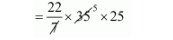# The diameters of the top and the bottom portions`
Question:

The diameters of the top and the bottom portions of a bucket are 42 cm and 28 cm respectively. If the height of the bucket is 24 cm, then the cost of painting its outer surface at the rate of 50 paise / cm2 is

(a) Rs. 1582.50

(b) Rs. 1724.50

(c) Rs. 1683

(d) Rs. 1642

Solution:

Radius of top of bucket $r_{1}=\frac{42}{2}=21 \mathrm{~cm}$

Radius of bottom of bucket $r_{2}=\frac{28}{2}=14 \mathrm{~cm}$

Height of bucket, $h=24 \mathrm{~cm}$.

$I=\sqrt{h^{2}\left(r_{1}-r_{2}\right)}$

$=\sqrt{576+(21-14)^{2}}$

$=\sqrt{576+49}$

$=\sqrt{625}$

$=25$

C.S.A. of the bucket

$=\pi\left(r_{1}+r_{2}\right) l$

$=\pi(21+14) \times 25$= 2750 cm2

Area of bottom

$=\pi r^{2}$

$=\frac{22}{7} \times 196$

$=616 \mathrm{~cm}^{2}$

The cost of painting its C.S. ,

$=(2750+616) \times \frac{1}{2}$

$=3366 \times \frac{1}{2}$

$=\operatorname{Rs} 1683$

Hence, the correct answer is choice (c).